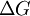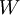# Jarzynski equalityThis article is a 'stub' page, it has no, or next to no, content. It is here at the moment to help form part of the structure of SklogWiki. If you add sufficient material to this article then please remove the {{Stub-general}} template from this page.
The Jarzynski equality is also known as the work relation or non-equilibrium work relation. According to it, the equilibrium free energy of a process,$\Delta G$, can be reconstructed by averaging the external work,$W$, performed in many nonequilibrium realizations of the process:$\exp \left( \frac{-\Delta G}{k_BT}\right)= \left\langle \exp \left( \frac{-W}{k_BT} \right) \right\rangle$